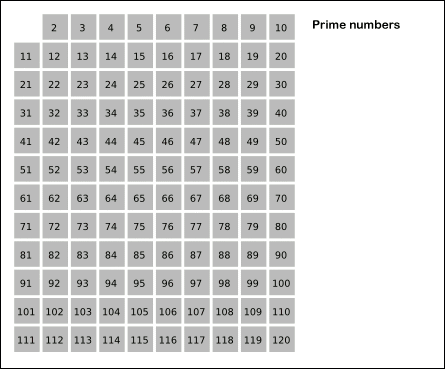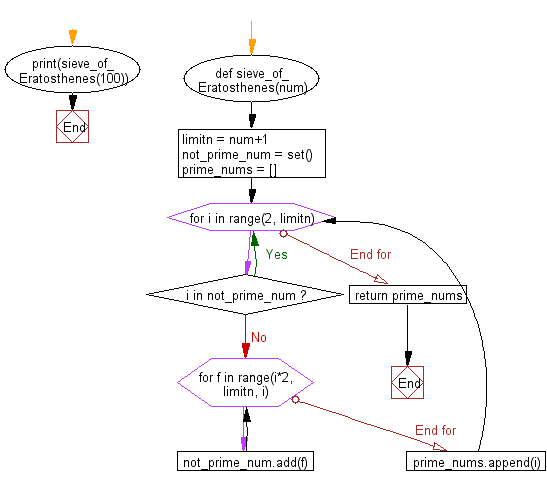﻿ Python Math: Print all primes (Sieve of Eratosthenes) smaller than or equal to a specified number - w3resource# Python Math: Print all primes (Sieve of Eratosthenes) smaller than or equal to a specified number

## Python Math: Exercise-21 with Solution

Write a Python program to print all primes (Sieve of Eratosthenes) smaller than or equal to a specified number.

In mathematics, the sieve of Eratosthenes, one of a number of prime number sieves, is a simple, ancient algorithm for finding all prime numbers up to any given limit. It does so by iteratively marking as composite (i.e., not prime) the multiples of each prime, starting with the multiples of 2.

Pictorial presentation:Animation Credits: Author information here

Sample Solution:-

Python Code:

``````
def sieve_of_Eratosthenes(num):
limitn = num+1
not_prime_num = set()
prime_nums = []

for i in range(2, limitn):
if i in not_prime_num:
continue

for f in range(i*2, limitn, i):

prime_nums.append(i)

return prime_nums

print(sieve_of_Eratosthenes(100));
```
```

Sample Output:

```[2, 3, 5, 7, 11, 13, 17, 19, 23, 29, 31, 37, 41, 43, 47, 53, 59, 61, 67, 71, 73, 79, 83, 89, 97]
```

Flowchart:## Visualize Python code execution:

The following tool visualize what the computer is doing step-by-step as it executes the said program:

Python Code Editor:

Have another way to solve this solution? Contribute your code (and comments) through Disqus.

What is the difficulty level of this exercise?

Test your Programming skills with w3resource's quiz.

﻿

```>>> students = [{'name': 'John', 'score': 98}, {'name': 'Mike', 'score': 94}, {'name': 'Jennifer', 'score': 99}]